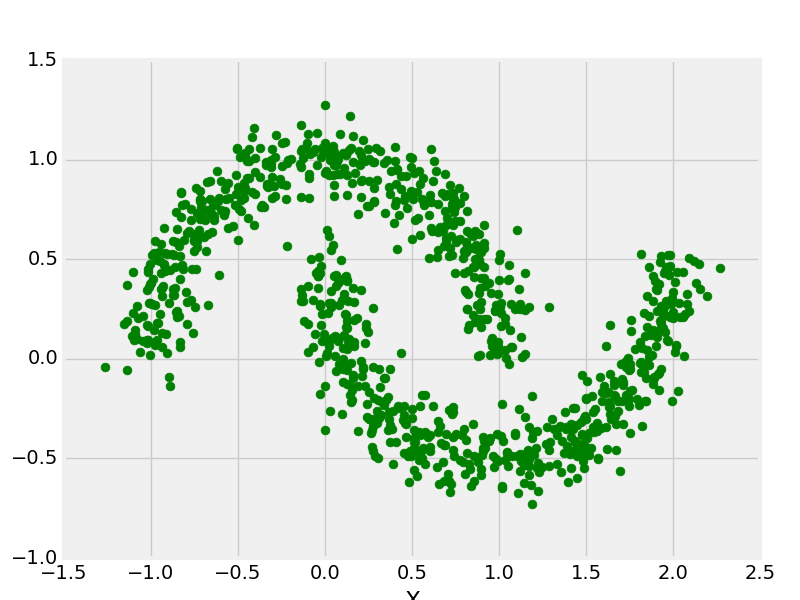GeeksforGeeks App
Open AppBrowser
Continue

# PyBrain – Importing Data For Datasets

In this article, we will learn how to import data for datasets in PyBrain.

Datasets are the data to be given to test, validate and train on networks. The type of dataset to be used depends on the tasks that we are going to do with Machine Learning. The most commonly used datasets that Pybrain supports are SupervisedDataSet and ClassificationDataSet. As their name suggests ClassificationDataSet is used in the classification problems and the SupervisedDataSet for supervised learning tasks.

## Method 1: Importing Data For Datasets Using CSV Files

This is the simplest method of importing any dataset from a CSV file. For this we will be using the Panda, so importing the Pandas library is a must.

Syntax: pd.read_csv(‘path of the csv file’)

Consider the CSV file we want to import is price.csv.

## Python3

 `import` `pandas as pd`` ` `print``(``'Read data...'``)`` ` `# enter the complete path of the csv file``df ``=` `pd.read_csv(``'../price.csv'``,header``=``0``).head(``1000``) ``data ``=` `df.values`

## Method 2:  Importing Data For DatasetsUsing Sklearn

There are many premade datasets available on the Sklearn library. Three main kinds of dataset interfaces can be used to get datasets depending on the desired type of dataset.

• The dataset loaders – They can be used to load small standard datasets, described in the Toy datasets section.

## Python3

 `from` `pybrain.datasets ``import` `ClassificationDataSet``from` `sklearn ``import` `datasets`` ` `nums ``=` `datasets.load_iris()``x, y ``=` `nums.data, nums.target``ds ``=` `ClassificationDataSet(``4``, ``1``, nb_classes``=``3``)``for` `j ``in` `range``(``len``(x)):``    ``ds.addSample(x[j], y[j])``ds`

Output:

<pybrain.datasets.classification.ClassificationDataSet at 0x7f7004812a50>

## Python3

 `from` `sklearn ``import` `datasets``from` `pybrain.datasets ``import` `ClassificationDataSet`` ` `digits ``=` `datasets.load_digits()``X, y ``=` `digits.data, digits.target``ds ``=` `ClassificationDataSet(``64``, ``1``, nb_classes``=``10``)``for` `i ``in` `range``(``len``(X)):``    ``ds.addSample(ravel(X[i]), y[i])`

Output:

<pybrain.datasets.classification.ClassificationDataSet at 0x5d4054612v80>

Example:

## Python3

 `import` `sklearn as sk``sk.datasets.fetch_california_housing`

Output:

<function sklearn.datasets._california_housing.fetch_california_housing>

• The dataset generation functions – They can be used to generate controlled synthetic datasets, described in the Generated datasets section. These functions return a tuple (X, y) consisting of a n_samples * n_features NumPy array X and an array of length n_samples containing the targets y.

Example:

## Python3

 `from` `sklearn.datasets ``import` `make_moon``from` `matplotlib ``import` `pyplot as plt``from` `matplotlib ``import` `style``  ` `X, y ``=` `make_moons(n_samples ``=` `1000``, noise ``=` `0.1``)``plt.scatter(X[:, ``0``], X[:, ``1``], s ``=` `40``, color ``=``'g'``)``plt.xlabel(``"X"``)``plt.ylabel(``"Y"``)``  ` `plt.show()``plt.clf()`

Output:My Personal Notes arrow_drop_up